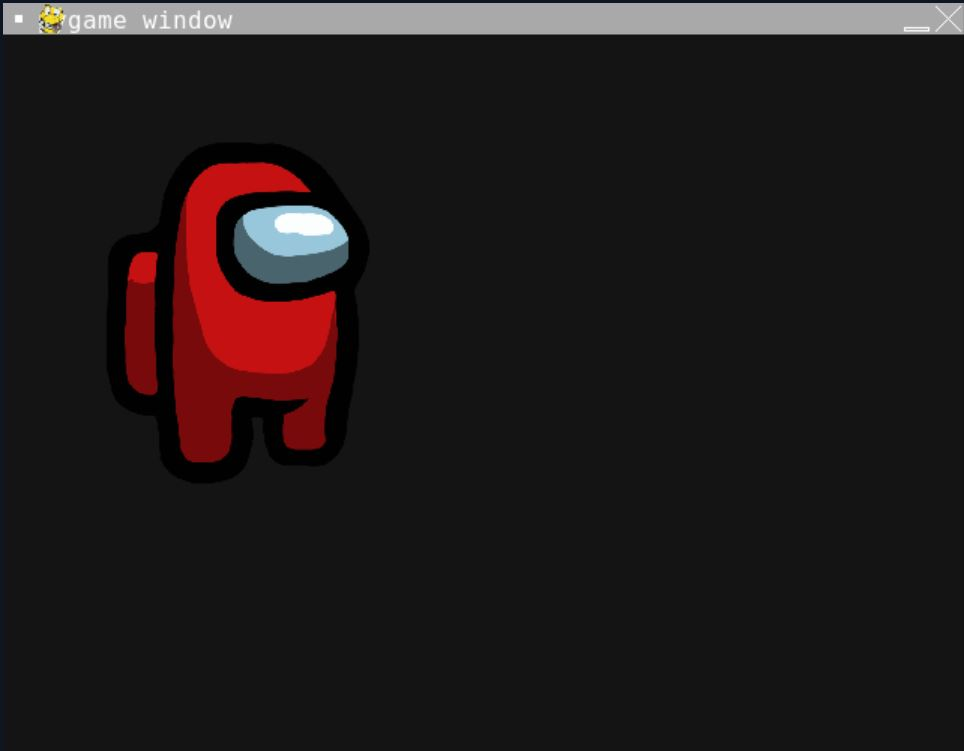# Scale Images in Pygame

Maxim Maeder May 11, 2022

This tutorial teaches you how to scale surfaces in Pygame.

Before we can transform the image, we need to import the `image.load()` method of Pygame. We will have to give it a path to an image and ensure that `jpg`, `png`, and `gif` are supported.

This function will return a new Surface which we already could blit onto the screen, but it could be that the image is too big or too small.

We will show you how to scale images in the next part.

``````image = pygame.image.load('img.jpg')
``````

## Scale Image in Pygame

We use the `pygame.transform.scale` function to scale an image. First, we must pass the surface on scaling and then the new width and height as an iterable (list or tuple).

This function will return the scaled surface. That’s why we overwrite the old surface.

This will stretch the image so it could look pretty distorted, so let’s now look at proportional scaling.

``````image = pygame.transform.scale(
image,
(width, height)
)
``````

## Scale Image Proportionally in Pygame

Pygame doesn’t provide a way to scale proportionally, but we can do this ourselves with the code you will find below. First, we import the image and save it to the image variable, and then we get the `rect` of the image surface with the `get_rect()` method.

This will return an array with the x and y position and the width and height. We splice it because we only need the width and height.

After that, we define a scale multiplicator to determine how smaller or larger the output image will be. In our case, it will be a third of the original size.

We continue by transforming the image, we first provide the surface to be scaled, and then we provide an array where we multiply the width and height with the size and turn both numbers into integers. The following snippet will scale it while preserving its ratio.

``````image = pygame.image.load('img.jpg')

ext = image.get_rect()[2:4]
size = 0.3

image = pygame.transform.scale(
image,
(int(ext*size), int(ext*size))
)
``````

Complete Code:

``````# Imports
import sys
import pygame

# Configuration
pygame.init()
fps = 60
fpsClock = pygame.time.Clock()
width, height = 640, 480
screen = pygame.display.set_mode((width, height))

ext = image.get_rect()[2:4]
size = 0.3

image = pygame.transform.scale(
image,
(int(ext*size), int(ext*size))
)

# Game loop.
while  True:
screen.fill((20, 20, 20))
for event in pygame.event.get():
if event.type == pygame.QUIT:
pygame.quit()
sys.exit()

screen.blit(image, (30, 30))

pygame.display.flip()
fpsClock.tick(fps)
``````

Output:Author: Maxim Maeder

Hi, my name is Maxim Maeder, I am a young programming enthusiast looking to have fun coding and teaching you some things about programming.

GitHub